## The Basics of Monte Carlo Simulations

#### University of Nebraska-Lincoln Physical Chemistry Lab (Chem 484) Written by Lab TA Joy Woller, Spring 1996

Summary: This document introduces the concept of Monte Carlo methods by defining the terms and describing a simple example (the determination of pi using a Monte Carlo simulation). Following this introduction is a section on the Monte Carlo experiment, part of the physical chemistry lab at UNL, which computes the population distribution in the rotational energy levels of HCl and DCl.

##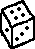Introduction to Monte Carlo Methods

The expression "Monte Carlo method" is actually very general. Monte Carlo (MC) methods are stochastic techniques--meaning they are based on the use of random numbers and probability statistics to investigate problems. You can find MC methods used in everything from economics to nuclear physics to regulating the flow of traffic. Of course the way they are applied varies widely from field to field, and there are dozens of subsets of MC even within chemistry. But, strictly speaking, to call something a "Monte Carlo" experiment, all you need to do is use random numbers to examine some problem.

The use of MC methods to model physical problems allows us to examine more complex systems than we otherwise can. Solving equations which describe the interactions between two atoms is fairly simple; solving the same equations for hundreds or thousands of atoms is impossible. With MC methods, a large system can be sampled in a number of random configurations, and that data can be used to describe the system as a whole.

"Hit and miss" integration is the simplest type of MC method to understand, and it is the type of experiment used in this lab to determine the HCl/DCl energy level population distribution. Before discussing the lab, however, we will begin with a simple geometric MC experiment which calculates the value of pi based on a "hit and miss" integration.

##Monte Carlo Calculation of Pi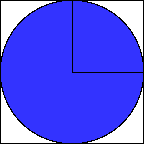Figure 1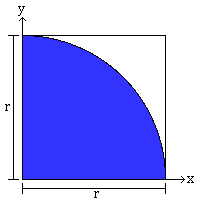Figure 2 Figure 1 is simply a unit circle circumscribed by a square. We could examine this problem in terms of the full circle and square, but it's easier to examine just one quadrant of the circle, as shown in Figure 2.  If you are a very poor dart player, it is easy to imagine throwing darts randomly at Figure 2, and it should be apparent that of the total number of darts that hit within the square, the number of darts that hit the shaded part (circle quadrant) is proportional to the area of that part. In other words,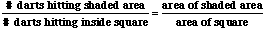If you remember your geometry, it's easy to show that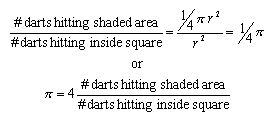If each dart thrown lands somewhere inside the square, the ratio of "hits" (in the shaded area) to "throws" will be four times the value of pi. If you actually do this experiment, you'll soon realize that it takes a very large number of throws to get a decent value of pi...well over 1,000. To make things easy on ourselves, we can have computers generate random* numbers.

If we say our circle's radius is 1.0, for each throw we can generate two random numbers, an x and a y coordinate, which we can then use to calculate the distance from the origin (0,0) using the Pythagorean theorem. If the distance from the origin is less than or equal to 1.0, it is within the shaded area and counts as a hit. Do this thousands (or millions) of times, and you will wind up with an estimate of the value of pi. How good it is depends on how many iterations (throws) are done, and to a lesser extent on the quality of the random number generator. Simple computer code for a single iteration, or throw, might be:

```	x=(random#)
y=(random#)
dist=sqrt(x^2 + y^2)
if dist.from.origin (less.than.or.equal.to) 1.0
let hits=hits+1.0
```

##Monte Carlo Computation of Population Distribution

The actual Monte Carlo method used in this lab to determine the population distribution among rotational energy levels is simpler than the two-dimensional example of the estimation of pi, as only one random number is generated for each "throw." This will be apparent shortly.

For this lab, the Boltzmann distribution can be solved analytically, and it is in fact used in determining the Monte Carlo distribution. As such, this is not a particularly informative simulation (you could just solve the Boltzmann equation for however many energy levels you wished and look at those numbers). However, this lab allows you to watch how changing the number of throws affects the results, and it automates the examination of the effects of temperature and isotope on population of energy levels.

The process used by the computer program for this lab is quite simple.

•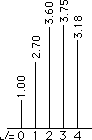• The input information is used to solve the Boltzmann equation for some number of energy levels. For a maximum of J=4, the relative populations might look like the figure to the right.
• The rest of the simulation is easier to understand if you imagine laying the peaks in the "spectrum" side-by-side, as shown below. If we generate random numbers along that line, whenever a number falls within the range of a particular J value, it counts as a "hit" for that energy level. Obviously, for shorter lengths (J=0) the number of "hits" will be smaller than for longer lengths (J=2).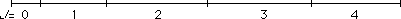• Since random number generators typically produce numbers on the range of zero to one, the population distribution is normalized so that the total "length" is equal to 1.0.
• For each "throw," or random number produced, the computer determines which energy level range it belongs to, and calls it a "hit" for that J value. After the computer completes all throws, the number of hits (or relative number of hits) for each energy level are given. It is up to you to compare this output to the theoretical distribution produced by the Boltzmann equation, as described in the Lab Report Instructions.

* Computer-generated numbers aren't really random, since computers are deterministic. But, given a number to start with--generally called a random number seed--a number of mathematical operations can be performed on the seed so as to generate unrelated (pseudorandom) numbers. The output of random number generators is tested with rigorous statistical tests to ensure that the numbers are random in relation to one another. One caveat: If you use a random number seed more than once, you will get identical random numbers every time. Thus, for multiple trials, different random number seeds must be used. Commercial programs, like Mathematica, pull a random number seed from somewhere within the system--perhaps the time on the clock--so the seed is unlikely to be the same for two different experiments.